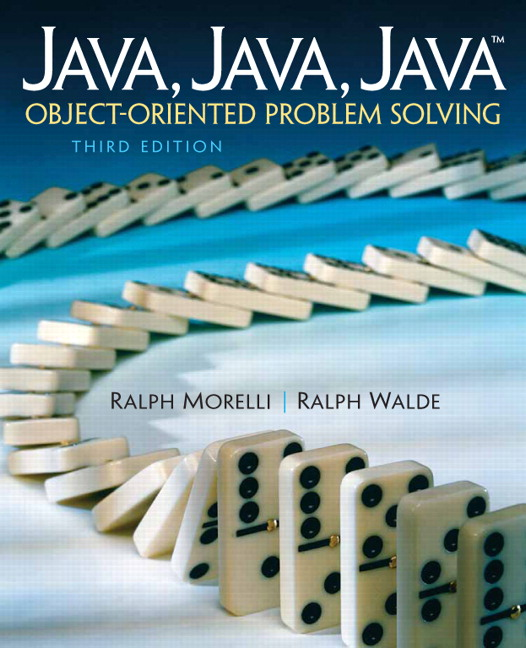# Java, Java, Java: Object-Oriented Problem Solving, 2022E

## Section6.4Example: Car Loan

Recall the self-study exercise from Chapter 5 that calculated the value of a bank CD (a) given its initial principle (p), interest rate (r), and number of years (n), using the formula a = p(1 + r)^n . This section explains how to use the same formula to calculate the cost of a car loan for different interest rates over various time periods.

### Subsection6.4.1Problem Description

For example, suppose you are planning on buying a car that costs $20,000. You find that you can get a car loan ranging anywhere from 8 to 11 percent, and you can have the loan for periods as short as two years and as long as eight years. Let’s use our loop constructs to create a table to show what the car will actually cost you, including financing. In this case, a will represent the total cost of the car, including the financing, and p will represent the price tag on the car ($20,000):
          8%          9%           10%         11%
Year 2  23,469.81   23,943.82   24,427.39   24,920.71
Year 3  25,424.31   26,198.42   26,996.07   27,817.98
Year 4  27,541.59   28,665.32   29,834.86   31,052.09
Year 5  29,835.19   31,364.50   32,972.17   34,662.19
Year 6  32,319.79   34,317.85   36,439.38   38,692.00
Year 7  35,011.30   37,549.30   40,271.19   43,190.31
Year 8  37,926.96   41,085.02   44,505.94   48,211.60


### Subsection6.4.2Algorithm Design

The key element in this program is the nested for loop that generates the table. Because the table contains seven rows, the outer loop can iterate seven times, through the values 2, 3, \dots 8 :
for (int years = 2; years <= 8; years++)

The inner loop should iterate through each of the interest rates, 8 through 11:
for (int years = 2; years <= 8; years++) {
for (int rate = 8; rate <= 11; rate++) {
} // for
} // for years

The financing calculation should be placed in the body of the inner loop together with a statement to print one cell (not row) of the table. Suppose the variable we use for a in the formula is carPriceWithLoan, and the variable we use for the actual price of the car is carPrice. Then our inner loop body is
carPriceWithLoan = carPrice *
Math.pow(1 + rate/100.0/365.0, years * 365.0);
System.out.print(dollars.format(carPriceWithLoan) +"\t");

Note that the rate is divided by both 100.0 (to make it a percentage) and by 365.0 (for daily compounding), and the year is multiplied by 365.0 before these values are passed to the Math.pow() method which returns the first argument to the power of the second argument. It is important here to use 100.0 and not 100 so that the resulting value is a double and not the int0.

### Subsection6.4.3Implementation

The program must also contain statements to print the row and column headings. Printing the row headings should be done within the outer loop, because it must be done for each row. Printing the column headings should be done before the outer loop is entered. Finally, our program should contain code to format the dollar and cents values properly. For this we use the java.text.NumberFormat class that was described in Chapter 5. The complete program is shown in Figure 6.4.1. Try it in the activecode below.

#### Activity6.4.1.

Try running the car loan program below. Change the amount of the loan and the interest rates in main and try it again.